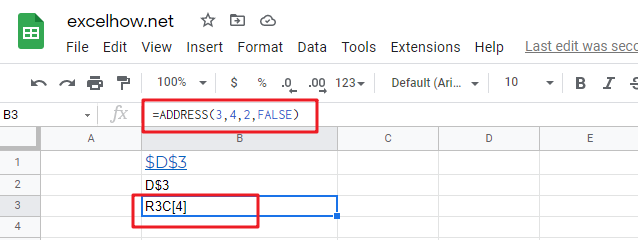This post will guide you how to use Google Sheets VLOOKUP function with syntax and examples.

## Description

The Google Sheets VLOOKUP function lookup a value in the first column of the table and return the value in the same row based on index_num position..

The VLOOKUP function can be used to search down the first column of a range for a key and returns the value of a specified cell in the row found in google sheets. The purpose of this function is to lookup a value in a table by matching on the first column and It’s returned value is the matched value from a table.

The VLOOKUP function is a build-in function in Google Sheets and it is categorized as a LOOKUP function.

## Syntax

The syntax of the VLOOKUP function is as below:

= VLOOKUP (lookup_value, table_array, column_index_num,[range_lookup])

Where the VLOOKUP function arguments are:

• Lookup_value -This is a required argument. The value that you want to search for, in the first column of the table. Note: the lookup_value can be a value, a cell or ranges reference, or a text string.
• Table_array – This is a required argument. Two or more columns of data to be searched in the first column.
• column_index_num – This is a required argument.  The column number in table_array.
• Range_lookup – This is an optional argument.  The value can be set as True or False. If true, the function will return an approximate match value. If False, it will find an exact match or it will return an error value #N/A.

Note:

• If range_lookup is TRUE, VLOOKUP function will match the nearest value less that the lookup_value.
• If range lookup is TRUE, VLOOKUP function will use an exact match if one exists.
• If range_lookup is FALSE, VLOOKUP function will perform an exact match.
• VLOOKUP function is not case-sensitive.
• If you want to search for numeric or data values, you need to make sure that the first column in the range is not sorted by text values.
• When range_lookup is set to TRUE and table_array is sorted, VLOOKUP function has much better performance.

## Google Sheets VLOOKUP Function Examples

The below examples will show you how to use google sheets VLOOKUP Function to lookup value from a table or array vertically.

#1 To search “39” text string in the first column and returns the value from column 2 that is in the same row, just using the following VLOOKUP formula:

=VLOOKUP(39,A1:C4,2,FALSE)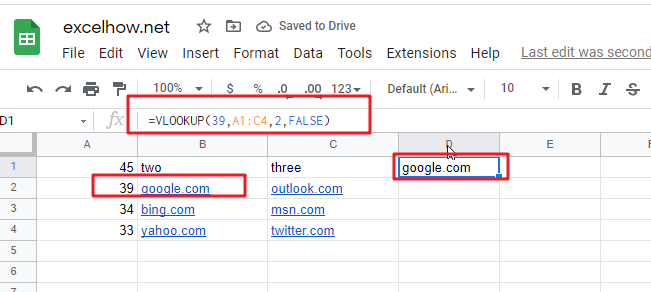This post will guide you how to use Google Sheets ROWS function with syntax and examples.

## Description

The Google Sheets ROWS function counts the number of rows in a cell reference.

The ROWS function can be used to return the number of rows in a specified range in google sheets. The purpose of this function is to get the number of rows in an array or cell reference and It’s returned value is a number of rows.

The ROWS function is a build-in function in Google Sheets and it is categorized as a LOOKUP function.

## Syntax

The syntax of the ROWS function is as below:

= ROWS(array)

Where the ROWS function arguments is:

• array-This is an required argument. An array, or A reference to a range of cells.

Note:

• ROWS just takes one argument, it can be a range or a array.
• Array can be a range or a cell reference
• If you want to count columns, you can use the COLUMNS function.
• If you want to get row numbers, you can use the ROW function.

## Google Sheets ROWS Function Examples

The below examples will show you how to use google sheets ROWS Function to return the number of rows in a range of cells.

#1 To get the number of rows in the cell range “A1:C4”, just using the following ROWS formula:

=ROWS(A1:C4)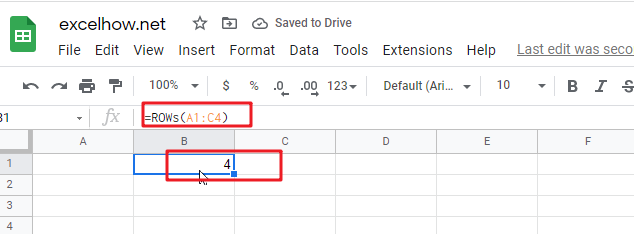This post will guide you how to use Google Sheets ROW function with syntax and examples.

## Description

The Google Sheets ROW function returns the row number of a cell reference.

The ROW function can be used return the row number of a specified cell in google sheets. The purpose of this function is to get the row number of a reference and It’s returned value is a number representing the row.

The ROW function is a build-in function in Google Sheets and it is categorized as a LOOKUP function.

## Syntax

The syntax of the ROW function is as below:

= ROW ([reference])

Where the ROW function argument is:

• Reference – This is an Optional argument. the cell or range of cells reference that you want to get the row number.

Note:

• If the reference is omitted, the ROW function will return the row number of the current cell.
• Reference can be a single cell address or a range of cells.
• Reference can not include multiple cell references.
• If you want to get column number, you can use the COLUMN function.
• If you want to count rows, you can use the ROWS function.

## Google Sheets ROW Function Examples

The below examples will show you how to use google sheets ROW Function to return the row number of a cell reference.
#1 To get the row number of “B5” cell in B1 Cell, just using the following ROW formula:

` =ROW(B5)`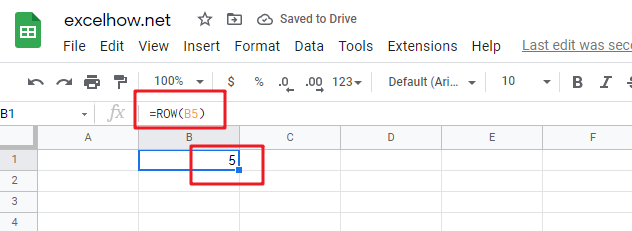#2 To get the row number of the current cell, just using below formula:

`=ROW()`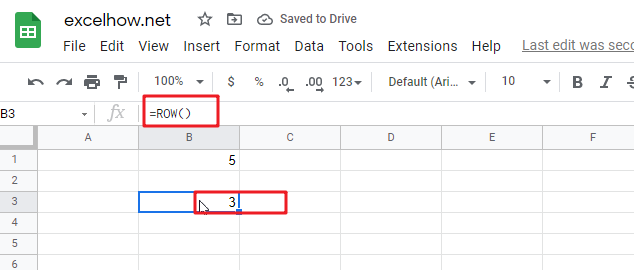#3 get the first row number of range in excel

You can get the first row number in a range with an formula based on the ROW function as follows:

```=ROW(Range)
=MIN(ROW(Range))```

The ROW function will only display the first row number.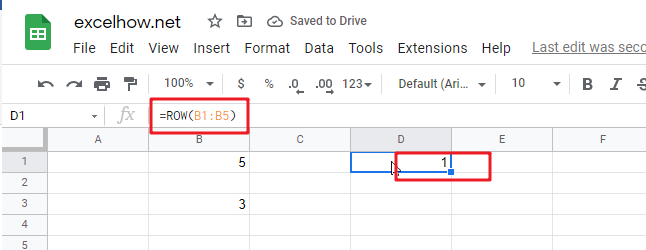This post will guide you how to use Google Sheets OFFSET function with syntax and examples. Most of google spreadsheet users are very confusing and don’t know how this function works in google sheets. After leaning this function, you should know that this function is very useful and you can read the below guide to understand how and why this function is very useful.

## Description

The Google Sheets OFFSET function returns a range reference shifted a specified number of rows and columns from a starting cell reference.

The OFFSET function can be used shift a range of a certain number of rows or columns in google sheets. The purpose of this function is to create a reference offset from a given starting cell reference and It’s returned value is a cell reference.

The OFFSET function is a build-in function in Google Sheets and it is categorized as a LOOKUP function.

## Syntax

The syntax of the OFFSET function is as below:

= OFFSET(cell_reference, offset_rows, offset_columns, [height], [width])

Where the OFFSET function arguments are:

• cell_reference -This is a required argument. The starting point from which to count the offset rows and columns.
• offset_rows – This is a required argument. The number of rows to offset to shift by.
• offset_columns – This is a required argument. The number of columns to offset to shift by.
• height – This is an optional argument. The height of the range to return starting at the offset target.
• width – This is an optional argument. The width of the range to return starting at the offset target.

Note:

• If offset_rows is a negative value, it is possible for the offset target to be outside of the spreadsheet. If this occurs, OFFSET function will return the #REF! error.
• If offset_columns is a negative value, it is possible for the offset target to be outside of the spreadsheet. If this occurs, OFFSET function will return the #REF! error.
• OFFSET function only returns a cell reference

## Google Sheets OFFSET Function Examples

The below examples will show you how to use google sheets OFFSET Function to shift a range of a certain number of rows or columns.

#1 =OFFSET(A1,3,1)

This OFFSET Formula will return the forth value in the second column, see the below screenshot: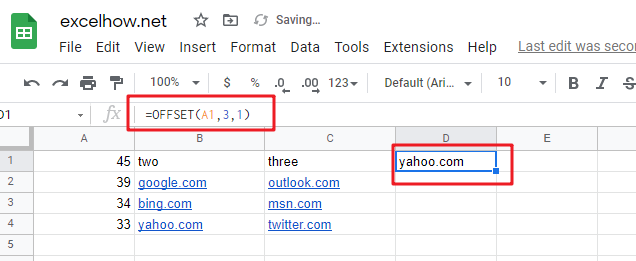This post will guide you how to use Google Sheets MATCH function with syntax and examples.

## Description

The Google Sheets MATCH function search a value in an array and returns the position of that item.

The MATCH function can be used to get the position of an item in the given range or array in google sheets. Its returned value is a number that representing a position in a range.

The MATCH function is a build-in function in Google Sheets and it is categorized as a LOOKUP function.

## Syntax

The syntax of the MATCH function is as below:

= MATCH(lookup_value, lookup_array, [match_type])

Where the MATCH function arguments are:

• Lookup_value -This is a required argument. The value that you want to search.
• Lookup_array – This is a required argument. The data array that is to be searched.
• Match_type – This is an optional argument. This value can be set as: 1, 0, -1. The default value is 1.

Note:

• – the MATCH function will search the largest value that is less than or equal to Lookup_value
• – The MATCH function will search the first value that is exactly equal to Lookup_value
• -1 – The Match function will search the smallest value that is greater than or equal to Lookup_value.
• MATCH function returns the position in an array or range of a matched value rather than the value itself. If you wish to return the value itself, and you need to use the INDEX function in combination with the MATCH function.

## Google Sheets MATCH Function Examples

The below examples will show you how to use google sheets MATCH Function to get the position of an item in a range.

#1 =MATCH(35,A1:A4,1)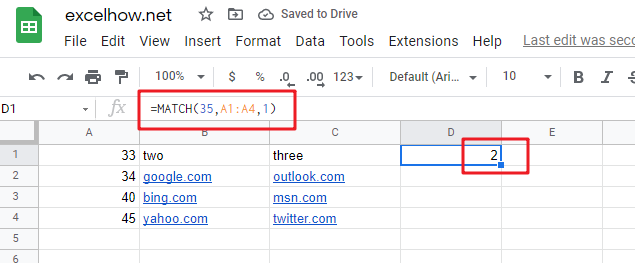This MATCH Formula will search the value “35” and find the largest value that is less than or equal to “35”, it will return “2”, As the value “34” is the only largest value that is less than to “35”. It will return the position of value “34” in range “A1:A4”.

#2 =MATCH(35,A1:A4,0)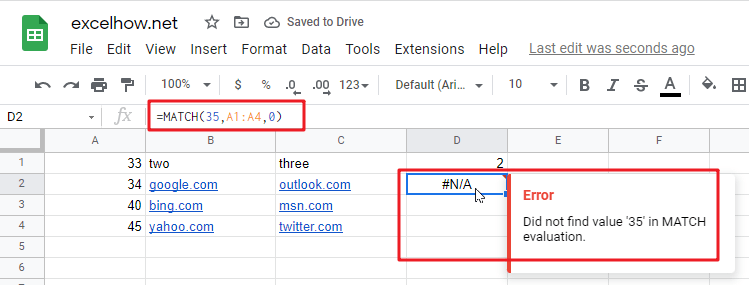This Formula will return the “#N/A” value, As the matched type is set to “0”, it means that the function will find the first value that is exactly equal to “35”, but it is not able to find the value “35” in “A1:A4” range.

#3 =MATCH(35,A1:A4,-1)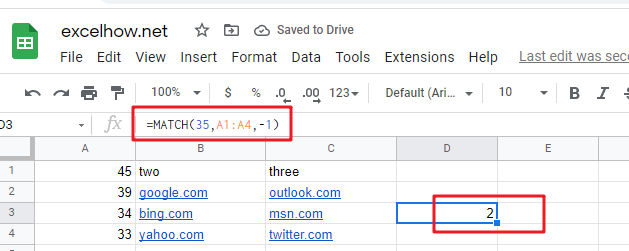This Formula will return “2”, if the Match type is set to “-1”, the Match function will find the smallest value that is greater than or equal to “35”in range “A1:A4”, then return its position.

This post will guide you how to use Google Sheets INDIRECT function with syntax and examples.

## Description

The Google Sheets INDIRECT function  returns the cell reference based on a text string, such as: type the text string “A2” in B1 cell, it just a text string, so you can use INDIRECT function to convert text string as cell reference.

The INDIRECT function can be used to get a valid cell reference from a given text string in google sheets. The purpose of this function is to get a cell reference from a text string, and the returned value is a valid cell reference.

The INDIRECT function is a build-in function in Google Sheets and it is categorized as a Lookup function.

## Syntax

The syntax of the INDIRECT function is as below:

= INDIRECT(ref_text,[a1])

Where the INDIRECT function arguments are:

• Ref_text -This is a required argument. A reference to a cell as a text string.
• A1 – This is an optional argument. It is a logical value that specify the style of the Ref_text reference.

Note:

• If A1 value is True or omitted, the ref_text will be interpreted as an A1-style reference.
• If A1 value is False, the ref_text will be interpreted as R1C1 style reference.

## Google Sheets INDIRECT Function Examples

The below examples will show you how to use google sheets INDIRECT Function to return a  cell or range reference that is represented by a text string.

#1 To get the value of the reference in cell A2, just using the following INDIRECT formula:

`=INDIRECT(A2)`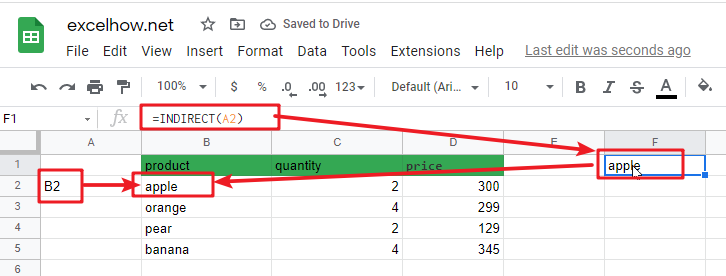This post will guide you how to use Google Sheets INDEX function with syntax and examples.

## Description

The Google Sheets INDEX function returns a value from a table based on the index (row number and column number). You can use INDEX function to extract entire rows or entire columns. This function is used to combine with the MATCH function to lookup value in a range or array.

The INDEX function can be used to extract the value at a given location in a range in google sheets. The purpose of this function is to get a value in a table based on a location, and the returned value is the value at a given location.

The INDEX function is a build-in function in Google Sheets and it is categorized as a Lookup function.

## Syntax

There are two ways to use the INDEX function in excel:

If you want to get the value of a specified cell or array of cell, you can refer to Array form.

If you want to get a reference to specified cells, you can refer to Reference form.

The syntax of the INDEX function is as below:

= INDEX (array, row_num,[column_num])      #Array form

=INDEX(reference, row_num,[column_num],[area_num)     #Reference form

The array form is used in most cases, and if the first argument of the INDEX function is an array constant, you need to use the array form. If you want to perform a three-way lookup in a range, you can use the reference form.

Where the INDEX function arguments are:

• array -This is a required argument. A range of cells or data array. If array contains only one row or column, the corresponding row_num or Column_num argument is optional.
• Row_num – The row number in data array. If Row_num is omitted, column_num is required.
• Column_num – The column position in data array. If Column_num is omitted, row_num is required.
• Area_num – it is set as a number. If the first argument point to more cell ranges, if area_num is set to 1, then the first area will be selected.

Note:

• If you set the row_num and column_num at the same time, the INDEX function will return the value in the cell at the intersection of row number and column number.
• If you set the value of row_num or column_num to 0, the INDEX function will return the array of values for the entire row or column in the array data.
• Row_num and Column_num must point to a cell within array data, if not, the index function returns #REF!

## Google Sheets INDEX Function Examples

The below examples will show you how to use google sheets INDEX function to return a value from a table based on the intersection of row number and column number.

#1 To get the value at the intersection of the second row and second column in the table array: A1:C2, just using the following INDEX formula:

`=INDEX(A1:C2,2,1)`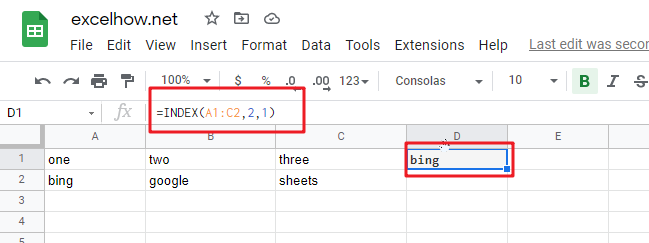This post will guide you how to use Google Sheets LOOKUP function with syntax and examples.

## Description

The Google Sheets LOOKUP function looks up a value through a sorted row or column and returns the corresponding value from another row or column.

The LOOKUP function can be used to lookup a value in a one-row or one-column range in google sheets.

The LOOKUP function is a build-in function in Google Sheets and it is categorized as a Lookup function.

## Syntax

The syntax of the LOOKUP function is as below:

= LOOKUP (lookup_value, lookup_vector, [result_vector])

Where the LOOKUP function arguments are:

• Lookup_value -This is a required argument. A value that you want to search in the lookup_vector.
• Lookup_vector – This is a required argument. A Range that only contains one row or one column.
• Result_vector – This is an optional argument. A Range that only contains one row or one column. It must be the same size as Lookup_vector. The lookup function will look up the value in the lookup_value range and returns the value from the same position in the result_vector.

Note:

• If `result_vector` is omitted, the Lookup function will return the first column data.
• LOOKUP function will only work If data in lookup_vector is sorted. And this function assumes that `lookup_vector` is sorted in ascending order.
• When `lookup_value` is not found, LOOKUP function will return the next smallest value in the same row or column.
• When `lookup_value` is greater than all values in `lookup_vector`, LOOKUP function will return the last value.
• When `lookup_value` is less than the first value in `lookup_vector`, LOOKUP function will return the `#N/A` error.
• Result_vector must be only a single row or column.

## Google Sheets LOOKUP Function Examples

The below examples will show you how to use google sheets LOOKUP Function to search a value from a vector or array.

#1 To look up the value “34” in A1:A2 range, then get the value from the same row or column in B1:B2 range, just using the following google sheets formula:

`=LOOKUP(34,A1:A2,B1:B2)`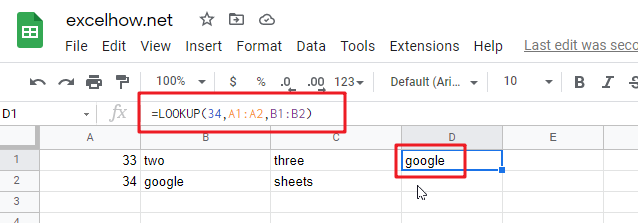This post will guide you how to use Google Sheets HLOOKUP function with syntax and examples.

## Description

The Google Sheets HLOOKUP function lookup a value in the top row of the table and return the value in the same column based on index_num position. The lookup values must be in the first row of the table. HLOOKUP function supports approximate and exact matching.

The HLOOKUP function can be used to lookup across the first row of a table for a keyword and returns the value of a cell in the same column found in google sheets. The purpose of this function is to look up a value in a table, and the returned value is the matched value from a given table.

The HLOOKUP function is a build-in function in Google Sheets and it is categorized as a Lookup function.

## Syntax

The syntax of the HLOOKUP function is as below:

= HLOOKUP (lookup_value, table_array, row_index_num,[range_lookup])

Where the HLOOKUP function arguments are:

• Lookup_value -This is a required argument. The value that you want to search for, in the first row of the table. Note: the lookup_value can be a value, a cell or ranges reference, or a text string.
• Table_array – This is a required argument. Two or more rows of data to be searched in the top row.
• Row_index_num – This is a required argument. The row number in table_array.
• Range_lookup – This is an optional argument. The value can be set as True or False. If true, the function will return an approximate match value. If False, it will find an exact match or it will return an error value #N/A.

## Google Sheets HLOOKUP Function Examples

The below examples will show you how to use google sheets HLOOKUP Function to retrieve a value from a horizontal data table.

#1 To search “two” text string in row 1 and returns the value from row 2 that is in the same column, just using the following formula:

`=HLOOKUP("two",A1:C2,2,FALSE())`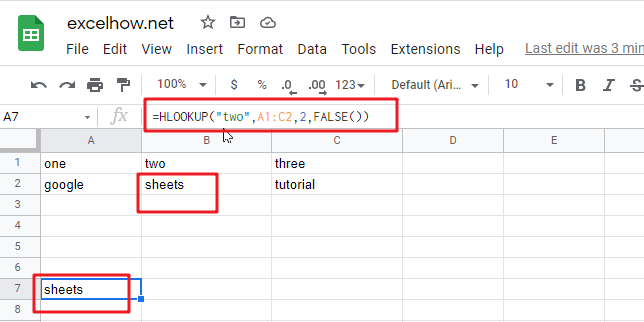This post will guide you how to use Google Sheets GETPIVOTDATA function with syntax and examples.

## Description

The Google Sheets GETPIVOTDATA function extracts specific data from a pivot table corresponds to the specified row and column name.

The GETPIVOTDATA function can be used to retrieve an aggregated value from a given pivot table by row or column name in google sheets. The purpose of this function is to extract data from a pivot table and the returned value is the data the you requested.

The GETPIVOTDATA function is a build-in function in Google Sheets and it is categorized as a Lookup function.

## Syntax

The syntax of the GETPIVOTDATA function is as below:

=GETPIVOTDATA(value_name, any_pivot_table_cell, [original_column, …], [pivot_item, …])

Where the GETPIVOTDATA function arguments are:

• Value_name -This is a required argument. The name of the value in the pivot table
• Any_pivot_table_cell – This is a required argument. A reference to a cell in the desired pivot table.
• Original_columnThis is an optional argument. The name of the column in the pivot table.
• Pivot_itemThis is an optional argument. The name of the row or column shown in the pivot table corresponding to Original_column that you want to get.

Note:

• Value_name must be enclosed in quotation marks or a cell reference to any cell that containing the text.

## Google Sheets GETPIVOTDATA Function Examples

The below examples will show you how to use google sheets GETPIVOTDATA Function to retrieve data from a pivot table.

#1 Assuming that you have a pivot table in your google sheets, and you wish to get total sales from it, you can use the following GETPIVOTDATA function:

`=GETPIVOTDATA(A16,A17)`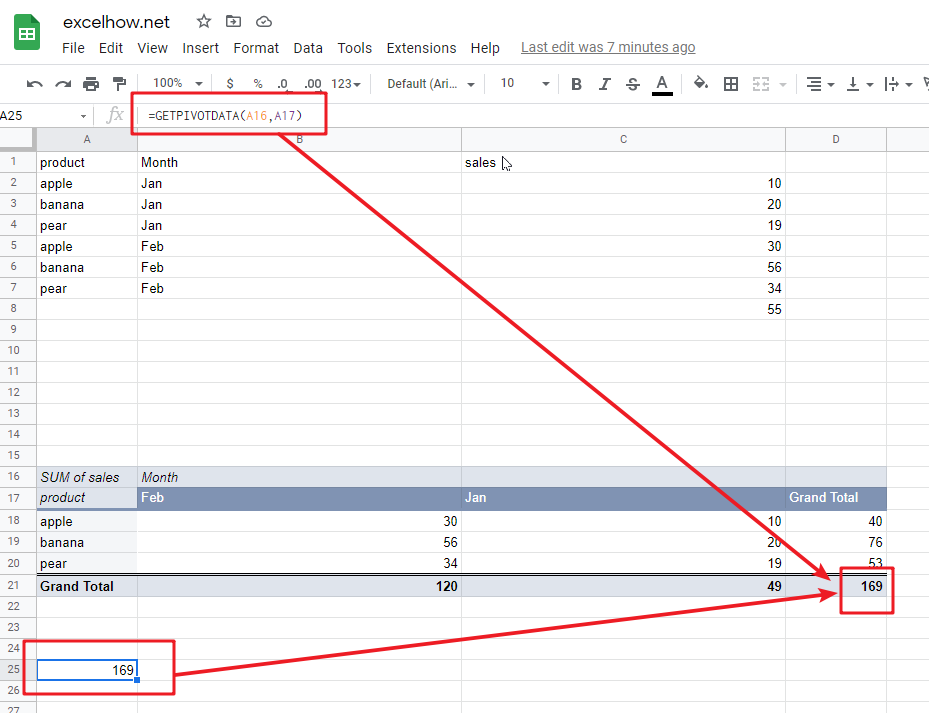This post will guide you how to use Google Sheets FORMULATEXT function with syntax and examples.

## Description

The Google Sheets FORMULATEXT function returns a formula as string. You can get a formula as a text string from a given cell reference.

The FORMULATEXT function can be used to extract the formula as text from a cell in google sheets. The purpose of this function is to get the formula in a cell and the returned value is the formula as text.

The FORMULATEXT function is a build-in function in Google Sheets and it is categorized as a Lookup function.

## Syntax

The syntax of the FORMULATEXT function is as below:

=FORMULATEXT (cell)

Where the FORMULATEXT function arguments are:

• cell -This is a required argument. The reference to cell or a cell range.

Note:

• FORMULATEXT function only take one arugument, it can be a cell reference.
• If Cell doesn’t contain a formula, the FORMULATEXT function returns #N/A error.
• FORMULATEXT function returns what is displayed in the formula bar when selecting a cell.
• If a cell range is passed into FORMULATEXT function, only the top left most cell is extracted.

## Google Sheets FORMULATEXT Function Examples

The below examples will show you how to use google sheets FORMULATEXT Function to extract a formula as a string.

#1 if the cell A1 contain one formula =NOW(), and you wish to extract this formula in cell A1 as a text string, just using the FORMULATEXT function:

`=FORMULATEXT(A1)`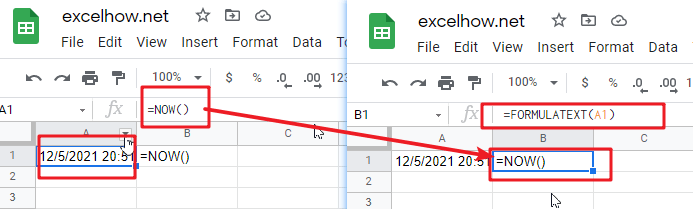#2 the cell A3 contain one string “google”, and using the FORMULATEXT function to extract a formula in Cell C3, it will returns #N/A error, type:

`=FORMULATEXT(C1)`#3 If the cell passed into FORMULATEXT references the cell that contains the FORMULATEXT formula, it can handle this kind of circular reference, type:

`=FORMULATEXT(B3)`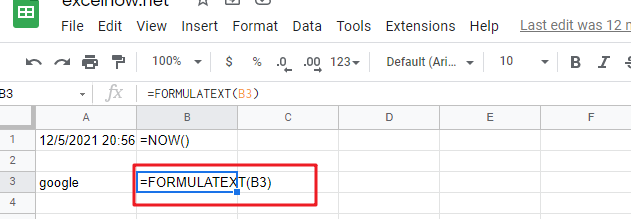This post will guide you how to use Google Sheets COLUMNS function with syntax and examples.

## Description

The Google Sheets COLUMNS function returns the number of columns in an Array or a reference. For example, COLUMNS(B1:D4) returns 3, since the range B1:D4 contains 3 columns.

The COLUMNS function can be used to get the count of columns in a given cell range in google sheets. The purpose of this function is to get the number of columns in an array range or cell reference and the returned value is a number that representing the COLUMNS.

The COLUMNS function is a build-in function in Google Sheets and it is categorized as a Lookup function.

## Syntax

The syntax of the COLUMNS function is as below:

=COLUMNS (array)

Where the COLUMNS function arguments are:

• Array -This is a required argument. A reference to a cell or a range of cells.

Notes:

• Array can be a cell range or a reference that containing a group of cells
• If you want to get column numbers, just see also COLUMN function.
• If you want to get row numbers, just see also ROW function.
• If you want to get the number of rows in given array or cell range, just see also ROWs function.

## Google Sheets COLUMNS Function Examples

The below examples will show you how to use google sheets COLUMNS to return the number of columns in a given range.

#1 To get the number of columns in the reference D1:F5, just using the following excel formula:

`=COLUMNS(D1:F5)`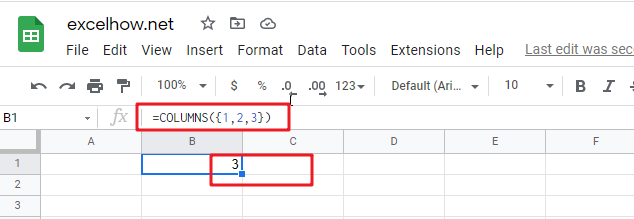#2 Using COLUMNS function to get a column count for a given array constant “{1,2,3}”, just using the following formula:

`=COLUMNS({1,2,3})`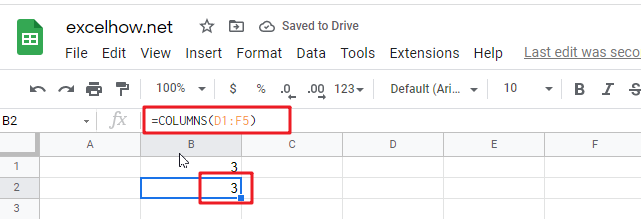This post will guide you how to use Google Sheets COLUMN function with syntax and examples.

## Description

The Google Sheets COLUMN function returns the first column number of the given cell reference. For example, COLUMN(B4) returns 2, as C is the second column in the google sheets.

The COLUMN function can be used to get the column number for a cell reference in google sheets. The purpose of this function is to get the column number of a reference and the returned value is a number that representing the column.

The COLUMN function is a build-in function in Google Sheets and it is categorized as a Lookup function.

## Syntax

The syntax of the COLUMN function is as below:

=COLUMN ([reference])

Where the COLUMN function arguments are:

• Reference -This is an optional argument. A reference to a cell or a range of cells for which you want to get the first column number.

Note:

• If the Array argument is omitted, the COLUMN function will return the column number of the cell that the function is entered in
• COLUMN function only takes one argument, it can be a cell reference, a cell range.
• COLUMN function can not contain multiple cell reference.

## Google Sheets COLUMN Function Examples

The below examples will show you how to use google sheets COLUMN Function to return the column number of a cell reference.

#1 To get the number of the column in B1 Cell, just using the following COLUMN formula:

`=COLUMN ( )`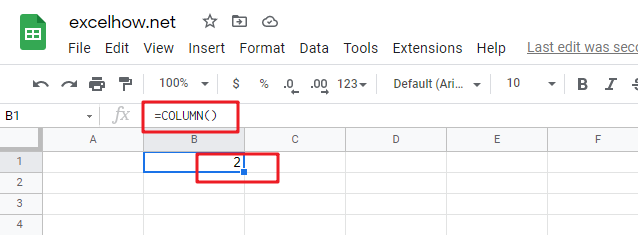#2 To get the number of column in the reference D1:F5, just using the following formula:

`=COLUMN(D1:F5)`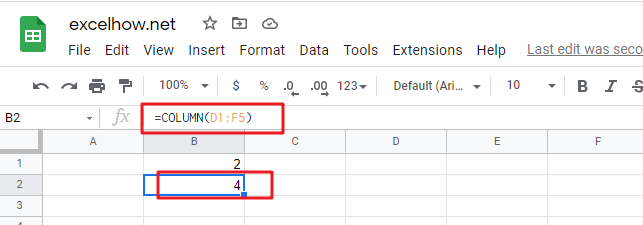This post will guide you how to use Google Sheets CHOOSE function with syntax and examples.

## Description

The Google Sheets CHOOSE function returns a value from a list of values based on index. For example, =CHOOSE(2,”google”,”sheets”,”book”) returns “sheets” value, and “sheets” value is the second value listed after the index number in this formula.

The CHOOSE function can be used to get a value from a list using a given index in google sheets. The purpose of this function is to get a value from a list based on a index and the returned value is the value at the given position.

The CHOOSE function is a build-in function in Google Sheets and it is categorized as a Lookup function.

## Syntax

The syntax of the CHOOSE function is as below:

=CHOOSE (index_num, value1,[value2],…)

Where the CHOOSE function arguments are:

• index_num -This is a required argument. Specify the position number in the list of values.
• Value1,[value2] – This is a required argument.  A list of one or more values that you want to return

Note:

• The index_num value must be a number between 1 and 29.
• If index_num value is less than 1 or greater than the length of the value list, the CHOOSE function will return #NUM!Error value.
• CHOOSE function will not retrieve values from a given range or array range
• Values can be an actual value or a cell reference.

## Google Sheets CHOOSE Function Examples

The below examples will show you how to use google sheets CHOOSE Function to return a value from a value list based on a position value.

#1 To get the second value in the value list in B1 Cell, just using the following formula:

`=CHOOSE(2,A1,A2,A3)`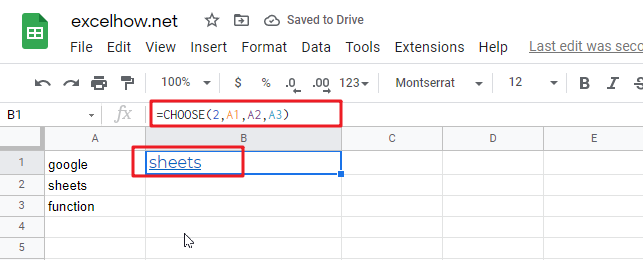#2 CHOOSE function will not retrieve values from a cell range, and it will return a #NUM! Error, like the below formula:

`=CHOOSE(2,A1:A3)`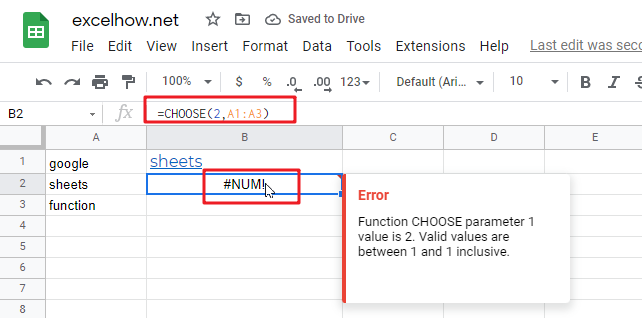#3 INDEX number is out of range in CHOOSE formula, it will retrun a #NUM! error, like:

`=CHOOSE(4,A1,A2,A3)`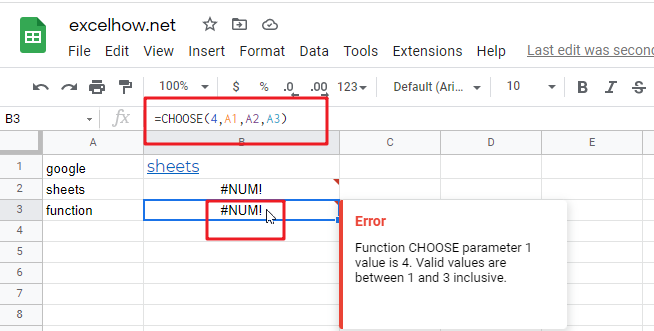This post will guide you how to use Google Sheets ADDRESS function with syntax and examples.

## Description

The Google Sheets ADDRESS function returns a reference as a text string to a single cell. For example, =ADDRESS(2,3) returns \$C\$2. The ADDRESS function can return a relative or absolute reference, and you can use it to construct a cell reference inside a formula.

The ADDRESS function can be used to get the address for a cell based on a given row and column number in google sheets. The purpose of this function is to create a cell reference from a row and column number and its returned value is a cell address.

The ADDRESS function is a build-in function in Google Sheets and it is categorized as a Lookup function.

## Syntax

The syntax of the ADDRESS function is as below:

=ADDRESS (row_num, column_num, [abs_num], [a1], [sheet_text])

Where the ADDRESS function arguments are:

• row_num -This is a required argument. The row number to use in the cell reference.
• column_num – This is a required argument. The column number to use in the cell reference.
• Abs_num – This is an optional argument. It will specify the type of reference to use. The following values can be used:

1 – Absolute

2 – Absolute row; relative column

3 – Relative row; absolute column

4 – Relative

• A1 – This is an optional argument. It will specify the style of reference to use.
• True– A1 reference style
• False – R1C1 reference style
• Sheet_text– This is a required argument. The sheet name to use.

The below examples will show you how to use google sheets ADDRESS function to return a reference as a text.

#1 Absolute reference, type the following formula:

`=ADDRESS(3,4)`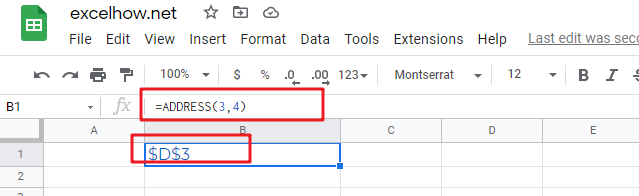#2 Absolute row, relative column, type the following formula:

`=ADDRESS(3,4,2)`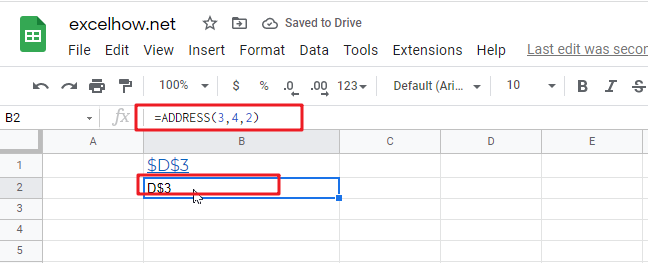#3 Absolute row, relative column in R1C1 reference style, type the following formula:

`=ADDRESS(3,4,2,FALSE)`